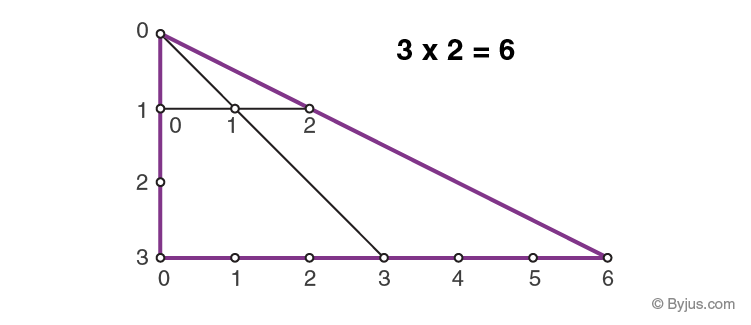# Additive Identity Vs Multiplicative Identity

In the world of Numbers which we deal with on daily basis, many of the properties of real numbers are used in operations such as addition, subtraction, multiplication, division etc. One such property which involves a specific operation on numbers resulting in obtaining the same number!! This property of numbers is called IDENTITY property. This article looks at this identity property and its characteristics.Additive identity of numbers, as the name suggests, is a property of numbers which is applied when carrying out addition operations. The property states that when a number is added to zero it will give the same number. “Zero” is called the identity element, (also known as additive identity) If we add any number with zero, the resulting number will be the same number. This is true for any real numbers, complex numbers and even for imaginary numbers.

Suppose, $a$ is any real number, then

$a ~+~ 0$ = $a$ = $0~ +~ a$

For example, $120 ~+~ 0$ = $120$ illustrates identity property of addition, where 0 is the additive identity.

## What is Multiplicative Identity?

Multiplicative identity of numbers, as the name suggests, is a property of numbers which is applied when carrying out multiplication operations Multiplicative identity property says that whenever a number is multiplied by the number $1$ (one) it will give that number as product. “$1$” is the multiplicative identity of a number. It is true if the number being multiplied is $1$ itself. The multiplicative identity property is represented as:

$a ~\times~ 1$ = $a$ = $1 ~\times~ a$  ($a$ is any real number)

Some examples:

$-1 ~+~ 0$ = $-1$ ($-1$ here is the number on which the operation is carried out and “$0$” is additive identity.

$0 ~+ ~259$      = $259$

$-1$ $\times$ $1$ = $-1$ ($-1$ here is the number on which the operation is carried out and “$1$” is multiplicative identity.

Note: $- 1$ $\times$ $-1$ = $1$ (proves that $-1$ is not a multiplicative identity)

### Additive and Multiplicative Identity Example

Example:

Which of the following illustrates the multiplicative identity and additive identity?

1. $45~ +~ 1$ = $46$
2. $50~ ×~ 2$ = $100$
3. $14~ × ~1$ = $14$
4. $-54 ~+~ 0$ = $-54$

Solution:

According to identity property of multiplication, the product of any number multiplied by $1$ is number itself.

Here, only $14$ $\times$ $1$ = $14$ satisfies the property.

Therefore, $14$ $\times$ $1$= $14$ illustrates the Multiplicative identity.

According to the identity property of addition, the sum of any number added to 0 is the number itself.

Here, only $-54~ +~ 0$ = $-54$ satisfied the property.

Therefore, $-54~ +~ 0$ = $-54$ illustrates the additive identity.

To solve more problems on the topic, download BYJU’S – The Learning App from Google Play Store and watch interactive videos. Also, take free tests to practice for exams. To study about other topics, visit BYJU’S and browse among thousands of interesting articles.